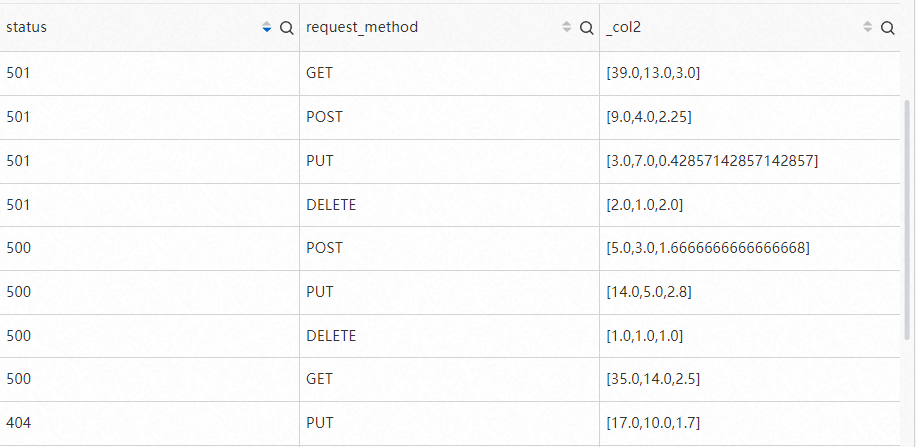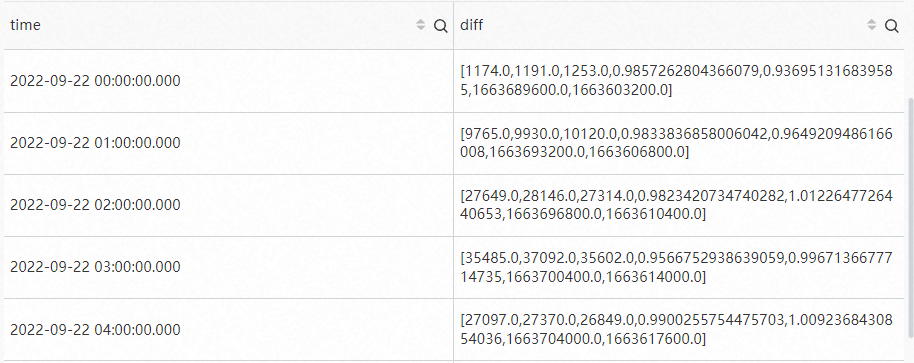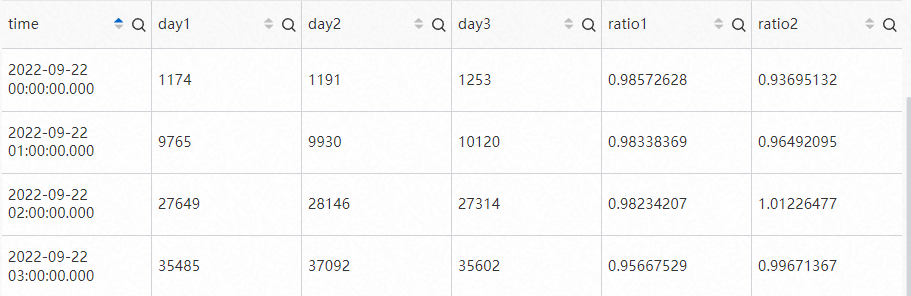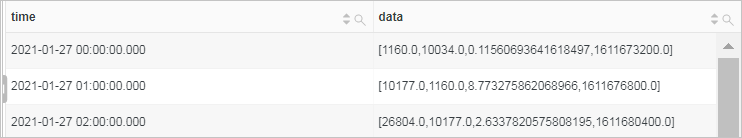This topic describes the syntax of interval-valued comparison functions and periodicity-valued comparison functions. This topic also provides examples on how to use the functions.

The following table describes the interval-valued comparison functions and periodicity-valued comparison functions that are supported by Log Service.

Important If you want to use strings in analytic statements, you must enclose the strings in single quotation marks (''). Strings that are not enclosed or strings that are enclosed in double quotation marks ("") indicate field names or column names. For example, 'status' indicates the status string, and status or "status" indicates the status log field.
FunctionSyntaxDescription
compare functioncompare(x, n)Compares the calculation result of the current time period with the calculation result of a time period n seconds before.
compare(x, n1, n2, n3...)Compares the calculation result of the current time period with the calculation results of multiple time periods n1, n2, and n3 seconds before.
ts_compare functionts_compare(x, n)Compares the calculation result of the current time period with the calculation result of a time period n seconds before.
ts_compare(x, n1, n2, n3...)Compares the calculation result of the current time period with the calculation results of multiple time periods n1, n2, and n3 seconds before.
Important
• When you use a compare function, the time based on which data is compared must be the same.

For example, you can use a compare function to compare the data of the current hour with the data of the same time period on the previous day. However, you cannot use a compare function to compare the data of the current hour with the data of the previous hour.

• The query and analysis results of the ts_compare function must be grouped by the time column by using the GROUP BY clause.

## compare function

The compare function compares the calculation result of the current time period with the calculation result of a time period n seconds before.

### Syntax

• Compare the calculation result of the current time period with the calculation result of a time period n seconds before.
``compare(x, n)``
• Compare the calculation result of the current time period with the calculation results of multiple time periods n1, n2, and n3 seconds before.
``compare(x, n1, n2, n3...)``

### Parameters

ParameterDescription
xThe value of this parameter is of the double or long type.
nThe time window. Unit: seconds. Examples: 3600, 86400, 604800, and 31622400. The values indicate 1 hour, 1 day, 1 week, and 1 year.

### Return value type

The array type. The return value is in the following format: [The calculation result of the current time period, The calculation result of a time period n seconds before, The ratio of the calculation result of the current time period to the calculation result of a time period n seconds before].

### Examples

• Example 1: Calculate the ratio of the page views (PVs) of the current hour to the PVs of the same time period on the previous day.

Set the time range for a query to 1 Hour(Time Frame) and execute the following query statement. 86400 specifies the current time minus 86,400 seconds, which is equivalent to one day. log specifies the name of the Logstore that is used.

• To display the query and analysis results in an array, execute the following query statement:
• Query statement
``````* |
SELECT
compare(PV, 86400)
FROM  (
SELECT
count(*) AS PV
FROM      log
)``````
• Query and analysis results• 3337.0 indicates the PVs of the current hour. Example: PVs from 14:00:00 to 15:00:00 on December 25, 2020.
• 3522.0 indicates the PVs of the same time period on the previous day. Example: PVs from 14:00:00 to 15:00:00 on December 24, 2020.
• 0.947473026689381 indicates the ratio of the PVs of the current hour to the PVs of the same time period on the previous day.
• To display the query and analysis results in multiple columns, execute the following query statement:
• Query statement
``````* |
SELECT
diff  AS today,
diff  AS yesterday,
diff  AS ratio
FROM  (
SELECT
compare(PV, 86400) AS diff
FROM      (
SELECT
count(*) AS PV
FROM          log
)
)``````

The result of the compare function is an array. In the preceding query statement, diff specifies the alias for the result, and diff  specifies the first value in the array.

• Query and analysis results• 3337.0 indicates the PVs of the current hour. Example: PVs from 14:00:00 to 15:00:00 on December 25, 2020.
• 3522.0 indicates the PVs of the same time period on the previous day. Example: PVs from 14:00:00 to 15:00:00 on December 24, 2020.
• 0.947473026689381 indicates the ratio of the PVs of the current hour to the PVs of the same time period on the previous day.
• Example 2: Query the numbers of request methods by request status of the current hour, and then compare the numbers of the current hour with the numbers of the same time period on the previous day.

Set the time range for a query to 1 Hour(Time Frame) and execute the following query statement. 3600 specifies the current time minus 3,600 seconds, which is equivalent to one day. log specifies the name of the Logstore that is used.

• Query statement
``````* |
SELECT
status,
request_method,
compare(PV, 3600)
FROM  (
SELECT
status,
request_method,
count(*) AS PV
FROM      log
GROUP BY
status,
request_method
)
GROUP BY
status,
request_method``````
• Query and analysis results## ts_compare function

The ts_compare function compares the calculation result of the current time period with the calculation result of a time period n seconds before.

Important The query and analysis results of the ts_compare function must be grouped by the time column by using the GROUP BY clause.

### Syntax

• Compare the calculation result of the current time period with the calculation result of a time period n seconds before.
``ts_compare(x, n)``
• Compare the calculation result of the current time period with the calculation results of multiple time periods n1, n2, and n3 seconds before.
``ts_compare(x, n1, n2, n3...)``

### Parameters

ParameterDescription
xThe value of this parameter is of the double or long type.
nThe time window. Unit: seconds. Examples: 3600, 86400, 604800, and 31622400. The values indicate 1 hour, 1 day, 1 week, and 1 year.

### Return value type

The array type. The return value is in the following format: [The calculation result of the current time period, The calculation result of a time period n seconds before, The ratio of the calculation result of the current time period to the calculation result of a time period n seconds before, The UNIX timestamp of a time period n seconds before].

### Examples

• Example 1: Calculate the ratio of the PVs of every hour today to the PVs of the same time period on the previous day and two days before.

Set the time range to Today(Time Frame) and execute the following query statement. 86400 specifies the current time minus 86,400 seconds, which is equivalent to one day. 172800 specifies the current time minus 172,800 seconds, which is equivalent to two days. log specifies the name of the Logstore that is used. date_trunc('hour',__time__ ) specifies that the return value is truncated by hour.

• To display the query and analysis results in an array, execute the following query statement:
• Query statement
``````* |
SELECT
time,
ts_compare(PV, 86400, 172800) as diff
FROM  (
SELECT
count(*) as PV,
date_trunc('hour', __time__) AS time
FROM      log
GROUP BY
time
)
GROUP BY
time
ORDER BY
time``````
• Query and analysis results• 1174.0 indicates the PVs of the current time period. Example: PVs from 00:00 to 01:00 on September 22, 2022.
• 1191.0 indicates the PVs of the same time period on the previous day. Example: PVs from 00:00 to 01:00 on September 21, 2022.
• 1253.0 indicates the PVs of the same time period two days before. Example: PVs from 00:00 to 01:00 on September 20, 2022.
• 0.9857262804366079 indicates the ratio of the PVs of the current time period to the PVs of the same time period on the previous day.
• 0.936951316839585 indicates the ratio of the PVs of the current time period to the PVs of the same period two days before.
• 1663689600.0 indicates the UNIX timestamp of 00:00 on September 21, 2022.
• 1663603200.0 indicates the UNIX timestamp of 00:00 on September 20, 2022.
Note The time returned in the query and analysis results varies based on the actual scenario.
• To display the query and analysis results in multiple columns, execute the following query statement:
• Query statement
``````* |
SELECT
time,
diff  AS day1,
diff  AS day2,
diff  AS day3,
diff  AS ratio1,
diff  AS ratio2
FROM  (
SELECT
time,
ts_compare(PV, 86400, 172800) AS diff
FROM      (
SELECT
count(*) as PV,
date_trunc('hour', __time__) AS time
FROM          log
GROUP BY
time
)
GROUP BY
time
ORDER BY
time
)``````
• Query and analysis results• Example 2: Calculate the ratios of the PVs of every hour to the PVs of the previous hour on the current day.

Set the time range to Today(Relative) and execute the following query statement. 3600 specifies the current time minus 3,600 seconds, which is equivalent to 1 hour. log specifies the name of the Logstore that is used. date_trunc('hour',__time__ ) specifies that the date_trunc function is used to truncate a time value by hour.

• Query statement
``````* |
SELECT
time,
ts_compare(PV, 3600) AS data
FROM(
SELECT
date_trunc('hour', __time__) AS time,
count(*) AS PV
FROM      log
GROUP BY
time
ORDER BY
time
)
GROUP BY
time``````
• Query and analysis resultsNote The time returned in the query and analysis results varies based on the actual scenario.